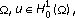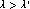# Eigenvalue Problems and Bifurcation of Nonhomogeneous Semilinear Elliptic Equations in Exterior Strip Domains

## Abstract

We consider the following eigenvalue problems:ininwhere,,is a smooth bounded domain,,is a smooth bounded domain insuch that. Under some suitable conditions onand, we show that there exists a positive constantsuch that the above-mentioned problems have at least two solutions if, a unique positive solution if, and no solution if. We also obtain some bifurcation results of the solutions at.

## References

1. Cao DM: Eigenvalue problems and bifurcation of semilinear elliptic equation in. Nonlinear Analysis. Theory, Methods & Applications 1995,24(4):529–554. 10.1016/0362-546X(94)E0071-N

2. Zhu XP: A perturbation result on positive entire solutions of a semilinear elliptic equation. Journal of Differential Equations 1991,92(2):163–178. 10.1016/0022-0396(91)90045-B

3. Cao DM, Zhou H-S: Multiple positive solutions of nonhomogeneous semilinear elliptic equations in. Proceedings of the Royal Society of Edinburgh. Section A 1996,126(2):443–463. 10.1017/S0308210500022836

4. Zhu XP, Zhou HS: Existence of multiple positive solutions of inhomogeneous semilinear elliptic problems in unbounded domains. Proceedings of the Royal Society of Edinburgh. Section A 1990,115(3–4):301–318. 10.1017/S0308210500020667

5. Esteban MJ: Nonlinear elliptic problems in strip-like domains: symmetry of positive vortex rings. Nonlinear Analysis. Theory, Methods & Applications 1983,7(4):365–379. 10.1016/0362-546X(83)90090-1

6. Lions P-L: The concentration-compactness principle in the calculus of variations. The locally compact case. I. Annales de l'Institut Henri Poincaré. Analyse Non Linéaire 1984,1(2):109–145.

7. Lions P-L: The concentration-compactness principle in the calculus of variations. The locally compact case. II. Annales de l'Institut Henri Poincaré. Analyse Non Linéaire 1984,1(4):223–283.

8. Bahri A, Lions P-L: On the existence of a positive solution of semilinear elliptic equations in unbounded domains. Annales de l'Institut Henri Poincaré. Analyse Non Linéaire 1997,14(3):365–413.

9. Lions P-L: On positive solutions of semilinear elliptic equations in unbounded domains. In Nonlinear Diffusion Equations and Their Equilibrium States, II (Berkeley, CA, 1986), Math. Sci. Res. Inst. Publ.. Volume 13. Edited by: Ni W-M, Peletier LA, Serrin J. Springer, New York, NY, USA; 1988:85–122.

10. Hsu T-S: Exactly two positive solutions of nonhomogeneous semilinear elliptic equations in unbounded cylinder domains. Dynamics of Continuous, Discrete & Impulsive Systems. Series A. Mathematical Analysis 2005,12(5):685–705.

11. Gilbarg D, Trudinger NS: Elliptic Partial Differential Equations of Second Order, Fundamental Principles of Mathematical Sciences. Volume 224. 2nd edition. Springer, Berlin, Germany; 1983.

12. Adams RA: Sobolev Spaces, Pure and Applied Mathematics. Volume 65. Academic Press, New York, NY, USA; 1975.

13. Hsu T-S: Multiple solutions for semilinear elliptic equations in unbounded cylinder domains. Proceedings of the Royal Society of Edinburgh. Section A 2004,134(4):719–731. 10.1017/S0308210500003449

14. Ekeland I: Nonconvex minimization problems. Bulletin of the American Mathematical Society 1979,1(3):443–474. 10.1090/S0273-0979-1979-14595-6

15. Graham-Eagle J: Monotone methods for semilinear elliptic equations in unbounded domains. Journal of Mathematical Analysis and Applications 1989,137(1):122–131. 10.1016/0022-247X(89)90276-X

16. Ambrosetti A, Rabinowitz PH: Dual variational methods in critical point theory and applications. Journal of Functional Analysis 1973,14(4):349–381. 10.1016/0022-1236(73)90051-7

17. Crandall MG, Rabinowitz PH: Bifurcation, perturbation of simple eigenvalues and linearized stability. Archive for Rational Mechanics and Analysis 1973,52(2):161–180.

18. Korman P, Li Y, Ouyang T: Exact multiplicity results for boundary value problems with nonlinearities generalising cubic. Proceedings of the Royal Society of Edinburgh. Section A 1996,126(3):599–616. 10.1017/S0308210500022927

19. Lien WC, Tzeng SY, Wang HC: Existence of solutions of semilinear elliptic problems on unbounded domains. Differential and Integral Equations 1993,6(6):1281–1298.

## Author information

Authors

### Corresponding author

Correspondence to Tsing-San Hsu.

## Rights and permissions

Reprints and Permissions

Hsu, TS. Eigenvalue Problems and Bifurcation of Nonhomogeneous Semilinear Elliptic Equations in Exterior Strip Domains. Bound Value Probl 2007, 014731 (2006). https://doi.org/10.1155/2007/14731